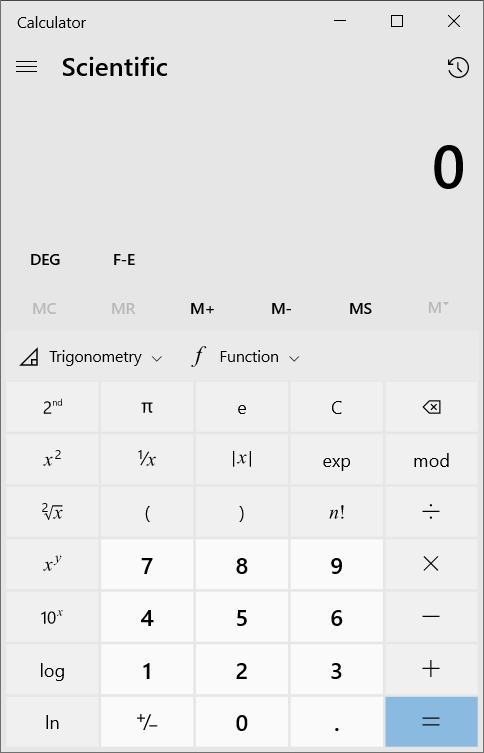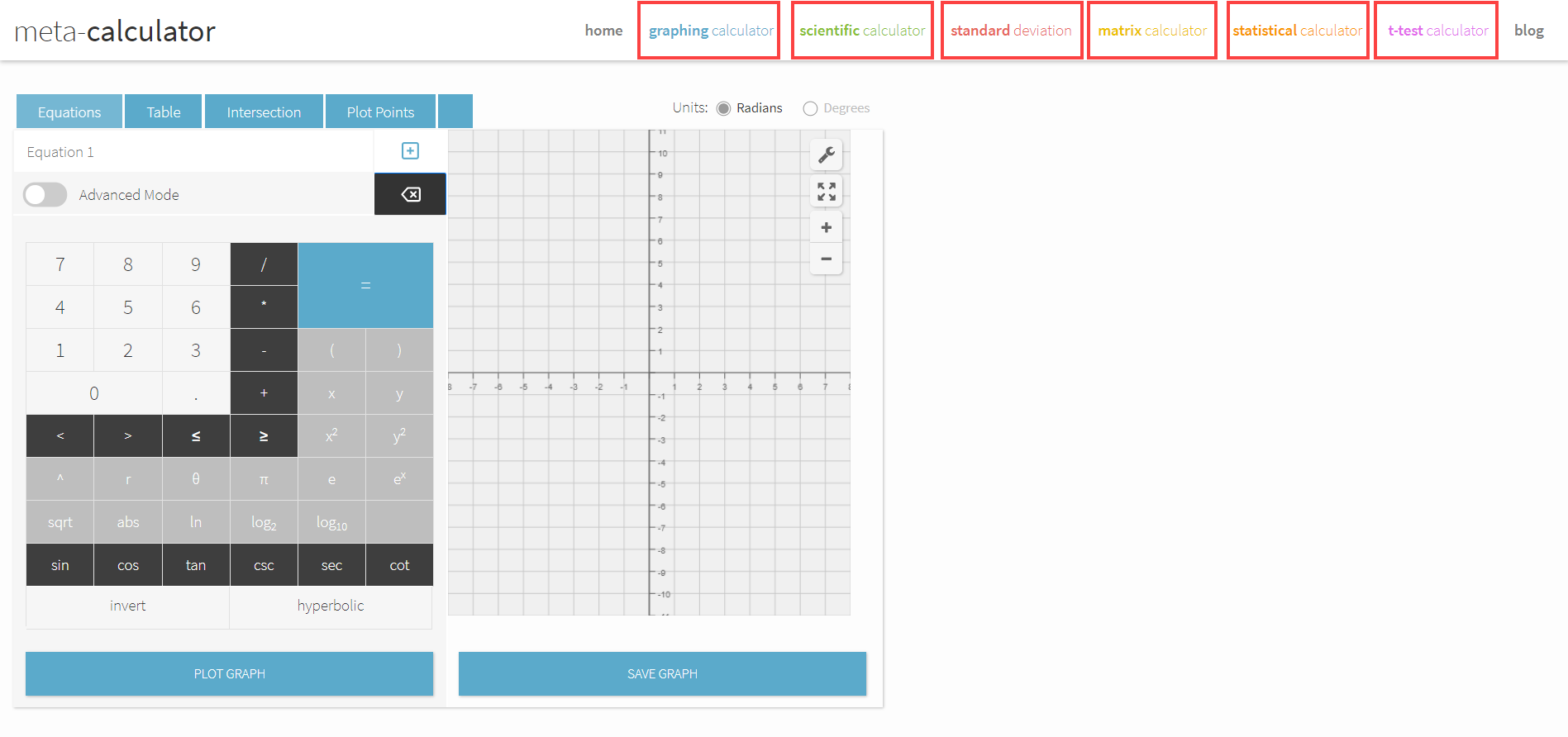# What Is A Scientific Calculator

Electronic calculators have been around for centuries, and they’ve gotten progressively better. However, the first scientific calculator was invented and released to the masses only decades ago, in 1968.

These devices are now a common sight in classrooms globally – but what exactly are they?

Furthermore, how are they different from basic calculators?

I’ve outlined the tool’s history, uses, special functions, and differences between regular and scientific calculators in this post to get you started with using the tool.

# What is a Scientific Calculator?

Scientific calculators are digital calculators loaded with functions that help solve science, engineering, and mathematics problems.

These calculators enable users to solve trigonometry problems using the embedded trigonometric, inverse, and hyperbolic functions. In addition, users can work with degrees, radians, and grads by simply switching modes, and mathematical constants like Pi and Euler’s constant come fed into these calculators for precise calculations.

The devices also enable working with exponents, logs, and scientific notations, making them the perfect tool for solving engineering problems.

The majority of these calculators available for purchase are handheld. However, these calculators weren’t portable until 1972, four years after they were first launched.

While Hewlett Packard was the first company to make scientific calculators available to the masses, Texas Instruments took control of a significant chunk of the market after releasing the SR-50 calculator in 1974. The TI-30 series remains one of the most popular series of scientific calculators to this day.

## Types of Scientific Calculators

There are four main types of scientific calculators: regular scientific calculators, graphing calculators, software-based calculators, and online scientific calculators.

Regular scientific calculators have a low-resolution screen and cannot plot coordinates.

Graphic calculators feature a high-resolution screen and enable users to graph functions and plot coordinates in a few taps.

Software-based calculators are embedded in most operating systems. For instance, the Windows 10 scientific calculator has most functions you need, as shown in the screenshot:Online calculators are, by far, the easiest to use. Meta-Calculator.com makes all the features available in expensive graphic calculators available for free. The best part about it is that you can access it from anywhere, at any time.## What is the Difference Between Scientific and Regular Calculators?

Besides scientific calculators, there are two other types of calculators: basic and business calculators.

The calculators can only perform basic functions – addition, subtraction, multiplication, and division. These calculators cannot perform operations like logarithms and work with exponents and trigonometric functions. h

Basic and business calculators also do not accept or output scientific notations and do not have any storage to save numbers. Storage is a critical feature for science and math classes since problems often have lengthy solutions, and calculated numbers may need to be used repeatedly.

Some other differences between scientific calculators and regular calculators include:

1. Scientific calculators have large, often high-resolution screens. Regular and business calculators have small screens.
2. Scientific calculators are capable of developing graphs of functions. However, simple calculators cannot perform much other than basic math.
3. Simple calculators are economical, while scientific calculators are expensive.
4. Scientific calculators follow operator precedence, whereas other calculators don’t — more on that below.

Let’s say you want to calculate 4 + 6 * 5. If you were to enter this expression into a simple calculator, the result would be 50.

However, if you enter the same expression into a scientific calculator, the answer would be 34 (the correct answer).

Scientific calculators work according to the order of operations – division is carried out first, multiplication next, addition thirdly, and subtraction in the end.

To get the correct answer with a regular/business calculator, you will need to enter parenthesis in the appropriate spots. This is not necessary when using scientific calculators.

## What Special Functions Do Scientific Calculators Have?

Most regular and business calculators have four or five functions at the most. In contrast, a scientific calculator may have dozens of functions.

While the functions included in scientific calculators vary by model and manufacturer, most scientific calculators can:

• Accept and output scientific notation;
• Work with floating-point arithmetic;
• Work with exponential functions;
• Give accurate root values beyond square roots;
• Work with trigonometric and also hyperbolic functions.

High-end scientific calculators come loaded with even more features, the most notable of which include:

• Working with complex numbers;
• Embedded cursor controls for easy editing of equations;
• Hexadecimal, binary, and octal number compatibility;
• Calculations with matrices;
• Calculus compatibility;
• Ability to do statistics and probability calculations.

Some scientific calculators are programmable, and some can also perform unit conversion (calculations like Celsius to Fahrenheit) and work with physical constants.

### Special Buttons

To enable users to perform the operations mentioned above, scientific calculators come with special buttons. The Casio FX-991EX ClassWiz is one of the most popular scientific calculators. Let’s look at its keys and how they work.You will see that the basic operations keys on this calculator are confined to the bottom. Above those keys, a pool of function keys enables the users to perform several critical operations.

The sin, cos, and tan keys make for easy trigonometric calculations. Pressing the SHIFT button allows the user to use the operation marked above each key.

Pressing the SHIFT key on the top-left of the calculator enables the user to work with inverse sin, cos, and tan functions.

The keys on the second row of the calculator enable working with fractions, finding square roots, squaring numbers, finding exponents of numbers, and much more.

Working with log base ten and base e is possible using the last two keys on the second row.

The calculator can perform several other functions – including working with scientific notations, finding inverses of numbers, and more.

The best way to find what a scientific calculator is capable of doing is to read its manual. You can download it from the internet before buying or read the physical copy that comes with the device.

## How to Use Special Keys?

To use the many functions that scientific calculators provide, you will need to get the hang of the correct way of entering the operations. In other words, you will need to understand which keys you must press before and after entering the number(s) you want to work with.

Here are some examples for you to understand better:

• Square root: Pressing the square root button after entering a number will result in a square root symbol appearing on the screen. For example, to calculate the square root of 9, you must press the square root button first and then feed the number 9 into the calculator.
• 10x: For you to find the value of 10 to the power of x, you will need to press the 10x button before entering the “x” value.
• x2: If you want to find the square of a number, you must enter the number you want to square before pressing the x2 button.
• x-1: The inverse function works similarly to the square function. You must enter the number you want to inverse first before pressing the button.
• Trigonometric functions: It is easy to understand that the operation must be punched in first before entering any numbers. What’s important to note is that scientific calculators allow you to work with radians and degrees.
• You can change in what form you want the answers to appear by navigating to the MODE menu and switching the option the calculator is set in. The indicator on the top of the screen will let you know what format you’re currently working with.
• Saving and restoring: As mentioned earlier, science and math problems can have lengthy solutions, and saving and restoring derived results becomes critical for solving them quickly. There are two ways to use calculator storage:
• You can use the “Answer” function to bring up the number/result that was last displayed on the screen. For instance, if you calculated the value of 3^3 first, you can enter “-10” into the calculator to subtract ten from the result.
• A more sophisticated way to store results is to arrive at the answer, press the STO button, then the ALPHA button, and press a key with a letter marked above it. Pressing the ENTER key will store the answer in the letter. You can then use the letter as a placeholder for that value.

## Conclusion

Learning to use a scientific calculator will help you burn through problems quickly – in practice and exams.

Now that you’ve understood what the tool is and how it works, you can try your hand at using it to solve problems. And the quickest and easiest way to do that is to use https://www.meta-calculator.com/.

We have various comprehensive calculators that you can use online for free. You can choose from t-test calculator, graphing, matrix, the standard deviation to statistics, and scientific calculators. Check it here